maths > construction-basics

Construction of Parallelograms

what you'll learn...

overview

In this page, constructing parallelograms is explained. It is outlined as follows.

•  Properties of parallelograms is explained

•  The number of independent parameters in a parallelogram is $3$$3$

•  For a given parameter, construction of parallelograms is approached as combination of triangles (sss, sas, asa, rhs, sal) and using the properties of parallelograms.

recap

A parallelogram is "a quadrilateral with two pair of parallel sides".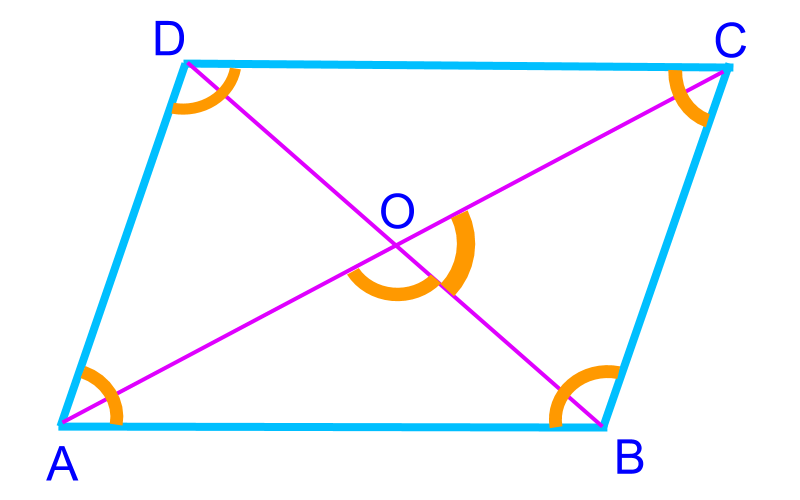Quadrilateral is defined by $5$$5$ parameters. In a parallelogram, the following properties provide dependency of parameters

•  opposite sides are parallel and that makes them equal

•  opposite angles are equal

•  diagonals bisect

•  two angles on diagonals are supplementary.

These properties cause two parameters to be dependent on other parameters and so, a parallelogram is defined by $3$$3$ parameters.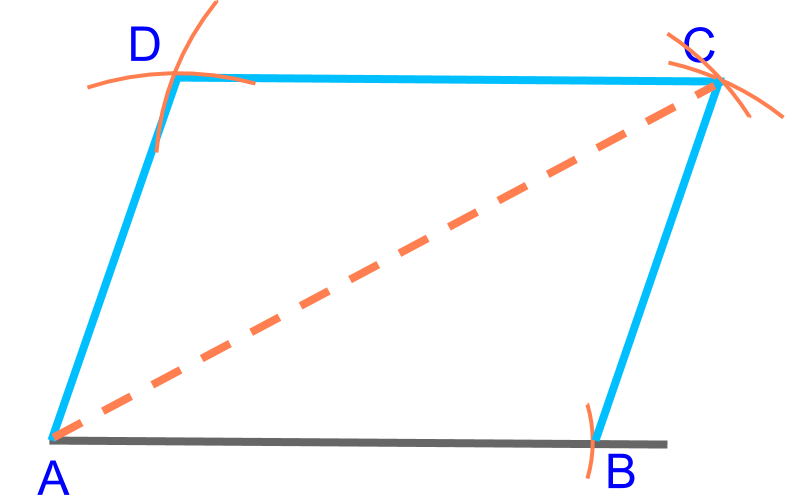To construct a parallelogram, $2$$2$ ($\overline{AB}$$\overline{A B}$, $\overline{BC}$$\overline{B C}$) sides and a diagonal ($\overline{AC}$$\overline{A C}$) are given. This is illustrated in the figure. To construct, Consider this as two SSS triangles $ABC$$A B C$ and $ACD$$A C D$.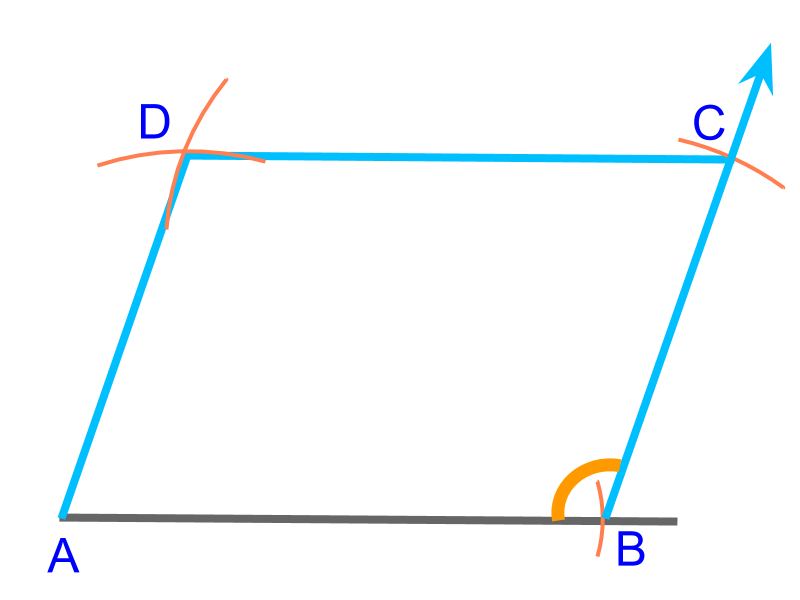To construct a parallelogram, $2$$2$ sides ($\overline{AB}$$\overline{A B}$, $\overline{BC}$$\overline{B C}$) and an angle ($\angle B$$\angle B$) are given. This is illustrated in the figure.

To construct the specified parallelogram, "Consider this as a SAS triangles $ABC$$A B C$ and another SSS triangle $ACD$$A C D$".

Note: Once the first SAS triangle $ABC$$A B C$ is completed, the $\overline{AC}$$\overline{A C}$ is fixed. Using that SSS triangle $ACD$$A C D$ is constructed.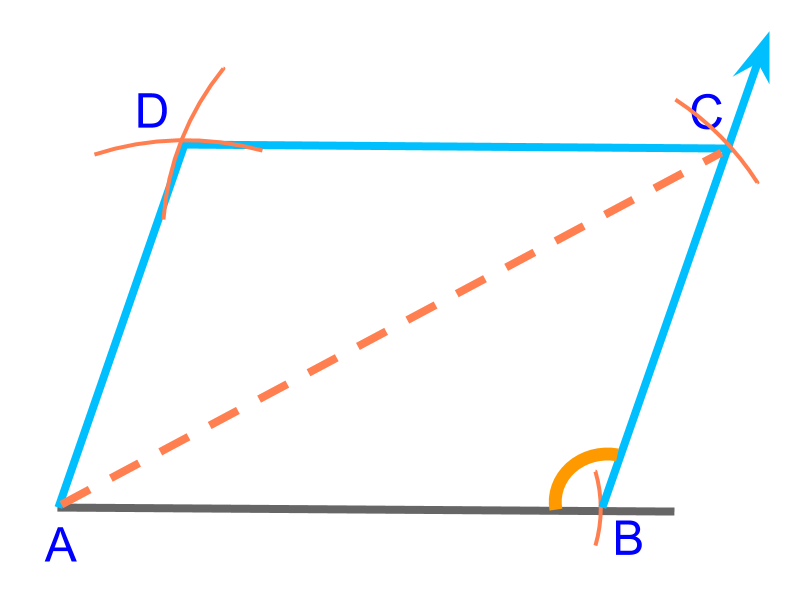To construct a parallelogram, a diagonal ($\overline{AC}$$\overline{A C}$), a side ($\overline{AB}$$\overline{A B}$), and an obtuse angle ($\angle B$$\angle B$) are given. This is illustrated in the figure. To construct the specified parallelogram "Consider this as an SSA triangles $ABC$$A B C$ and an SSS triangle $ACD$$A C D$".

Note: Once the first SAS triangle $ABC$$A B C$ is completed, that triangle can be copied to a SSS triangle $ACD$$A C D$.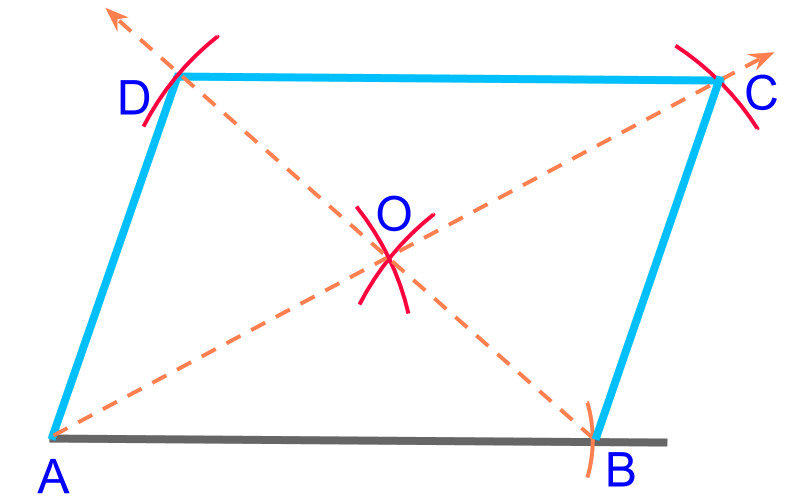To construct a parallelogram, a side ($\overline{AB}$$\overline{A B}$), and two diagonals ($\overline{AC}$$\overline{A C}$, $\overline{BD}$$\overline{B D}$) are given. This is illustrated in the figure.

To construct the specified parallelogram, "Consider this as an SSS triangles $AOB$$A O B$. Then construct points $C$$C$ and $D$$D$".

Note: The diagonals bisect, and $AOB$$A O B$ is constructed with half-diagonals. The $\stackrel{\to }{AO}$$\vec{A O}$ and $\stackrel{\to }{BO}$$\vec{B O}$ are extended. The half diagonals are marked from point $O$$O$ to construct vertices $C$$C$ and $D$$D$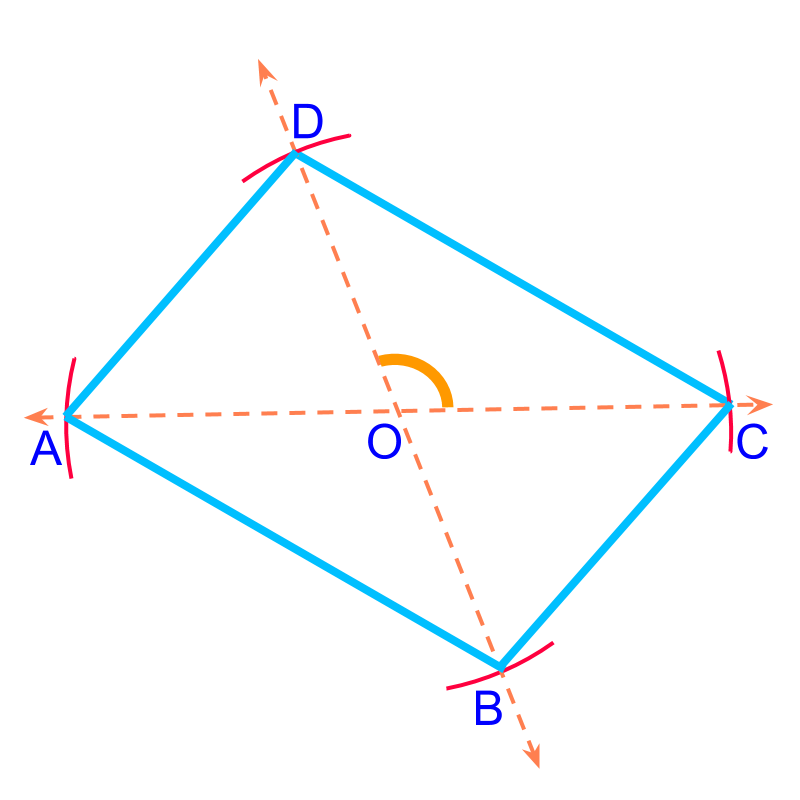To construct a parallelogram, two diagonals ($\overline{AC}$$\overline{A C}$, $\overline{BD}$$\overline{B D}$) and the angle between diagonals ($\angle AOB$$\angle A O B$) are given. This is illustrated in the figure.

To construct the specified parallelogram, "Consider this as two SAS triangles $DOC$$D O C$ and $AOB$$A O B$".

Note: Draw line $AOC$$A O C$ where points $A$$A$ and $C$$C$ are marked with half diagonal from point $O$$O$. At the given angle line $BOD$$B O D$ is drawn and points $B$$B$ and $D$$D$ are marked.

summaryConstruction of Parallelograms :

Properties of Parallelograms

•  opposite sides are parallel and equal

•  opposite angles are equal

•  diagonals bisect

•  two angles on diagonals are supplementary

The formulations of questions

•  $2$$2$ sides and $1$$1$ diagonal

•  $2$$2$ sides and $1$$1$ angle

•  $1$$1$ side, 1 diagonal and $1$$1$ angle

•  $1$$1$ side and $2$$2$ diagonals

•  $2$$2$ diagonals and $1$$1$ angle between diagonals

use properties to figure out dependent parameters and look for triangles

Outline